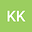Positive almost periodic solutions of nonautonomous evolution equations and application to Lotka--Volterra systems
•## Abstract

Consider the nonautonomous semilinear evolution equation of type: $(\star) \; u’(t)=A(t)u(t)+f(t,u(t)), \; t \in \mathbb{R},$ where $A(t), \ t\in \mathbb{R}$ is a family of closed linear operators on a Banach space $X$, the nonlinear term $f$, acting on some real interpolation spaces, is assumed to be almost periodic only in a weak sense (i.e. in Stepanov sense) with respect to $t$ and Lipschitzian in bounded sets with respect to the second variable. We prove the existence and uniqueness of positive almost periodic solutions in the strong sense (Bohr sense) for equation $(\star)$ using the exponential dichotomy approach. Then, we establish a new composition result of Stepanov almost periodic functions by assuming only the continuity of $f$ in the second variable. Moreover, we provide an application to a nonautonomous system of reaction–diffusion equations describing a Lotka–Volterra predator–prey model with diffusion and time–dependent parameters in a generalized almost periodic environment.
26 Feb 2022Submitted to Mathematical Methods in the Applied Sciences
01 Mar 2022Submission Checks Completed
01 Mar 2022Assigned to Editor
10 Mar 2022Reviewer(s) Assigned
21 Aug 2022Review(s) Completed, Editorial Evaluation Pending
27 Aug 2022Editorial Decision: Revise Major